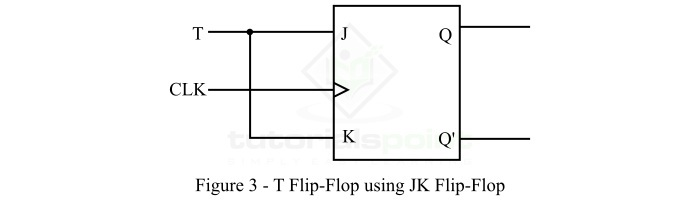# Conversion of JK Flip-Flop into T Flip-Flop

## What is a JK Flip-Flop?

JK flip flop is also a 1-bit storage device having two inputs similar to SR flip flop, but it has inputs denoted by J and K instead of S and R. It has two outputs viz. Q (normal output) and Q' (inverted output). The clock signal is used for synchronization of the circuit.

The block diagram of the JK flip flop is shown in Figure-1 below.The operation of the JK flip flop can be understood with the help of its truth table which is given below −

Inputs Output
J K Qn+1
00Qn
010
101
11Toggle

The characteristic equation of the JK flip flop is given by,

$$Q_{n+1}=JQ_{n}^{'}+K'Q_{n}$$

## What is a T Flip-Flop?

T flip-flop, also called toggle flip flop, is a type of digital flip flop which has a single input specified by the letter T. It has two outputs namely Q and Q', where Q is the normal output and Q' is the complemented output.

The block diagram of T flip flop is shown in Figure-2.The truth table of the T flip flop is depicted below.

Input (T) Present State of Output

(Qn)

Next State of Output

(Qn+1)

000
011
101
110

From the truth table of the T flip flop, it is clear that when the input T is equal to 0, there is no change in the output of the flip flop. When T is equal to 1, the output of the T flip flop toggles.

The characteristics equation of the T flip flop is given by,

$$Q_{n+1}=T'Q_{n}+TQ_{n}^{'}$$

After discussing the basics of JK flip flop and T flip flop, let us now discuss the conversion of JK flip flop into T flip flop.

## Conversion of Flip-Flop

We can convert a one type of flip-flop into another type of flip-flop. The conversion process of flip-flops involves the following steps −

• Step 1 − Write the excitation table of the flip flops.

• Step 2 − Simplify the excitation table with the help of Karnaugh Map (K-map).

• Step 3 − Draw the required logic circuit diagram.

Now, let us convert the JK flip-flop into the T flip-flop.

## Conversion of JK Flip-Flop into T Flip-Flop

The conversion of JK flip-flop into T flip-flop is done as per the following steps −

Step 1 − The excitation table for the conversion of JK flip flop to T flip flop is given below.

Input Present State of Output Next State of Output Flip Flop Inputs
TQnQn+1JKJK
000000X
0 1
0 1 1 0 0 X 0
1 0
1 0 1 1 0 1 X
1 1
1 1 0 0 1 X 1
1 1

Step 2 − The excitation table is simplified by using K-Map technique to obtain the expression of inputs as follows −

The K-map simplification for the input J is,J = T

The K-map simplification for the input K is,K = T

Step 3 − The logic diagram of the T flip-flop using JK flip flop is shown in Figure-3 below.In this way, we can convert JK flip flop into T flip flop.

Updated on: 24-Apr-2023

1K+ Views